#### You may also like### Days and Dates

Investigate how you can work out what day of the week your birthday will be on next year, and the year after...### Summing Consecutive Numbers

15 = 7 + 8 and 10 = 1 + 2 + 3 + 4. Can you say which numbers can be expressed as the sum of two or more consecutive integers?### Paving Paths

How many different ways can I lay 10 paving slabs, each 2 foot by 1 foot, to make a path 2 foot wide and 10 foot long from my back door into my garden, without cutting any of the paving slabs?

# Pocket Money

##### Age 11 to 14Challenge Level

Without doing any working out, different people chose different options.

Ellie and Eric from Dulwich College Seoul in South Korea, Rohan from Fen Ditton Primary School in the UK, Emma from Newton Poppleford Primary in the UK and Aneeka and Zara from North London Collegiate School in the UK would choose option 1. Ellie explained why:

I chose the first choice because the number was bigger than the others. It looked easier to get more money in allowance when the same big number repeated than when little numbers added to each other and made a total altogether.

Clara from Bangkok Patana School in Thailand chose option 2:
At first I thought that the second option would give the most money after 31 days because it would eventually increase to over 10 pounds (so it would be higher that option 1) and option 3 seemed like the start point was so small that it would have increased by very little by the end of a month

Tushar from West Island School in Hong Kong, Anana and Joshua from Dulwich College Seoul, Navjot from Sherborne Qatar, Devin from Takapuna Normal Intermediate School in New Zealand, Kazuma and Luanne from Bangkok Patana School, Mathieu, Maxim and Valentina from International School of Luxembourg, Annie and Anjeli from Harrow International School Hong Kong, Siya from North London Collegiate School, Joe and James from Walton High School in the UK and Ben from Kings Academy Ringmer in the UK all chose option 3. Anana said:
The option that I would chose would be the third option (doubling each day). This is because, I know that eventually the double of one of the numbers (on one of the days of the month) would be much bigger than the sum of the pocket money (at the end of the month) if I chose the other options.

James said:
Before starting to work out the problem, I decided option 3 was probably the best as your amount of daily pocket money grows exponentially as opposed to linearly. This means that the amount it is increasing by gets bigger each day and will eventually surpass option 2 which increases by a flat amount. Due to this property, it means the amount of pocket money I get every day will be larger than option 1 or 2 after a while.

Victoria K & F from Peak School in Hong Kong, Ally from Dulwich College Seoul, Jack, Keeli, Ethan and Ayaan from Fen Ditton Primary School, Napat and Shawn from Thailand, Thomas from Hollinwood Academy in the UK, Miranda from Redmaids' High School in the UK, Ahaan from TTS in Singapore, Tushan, Rohan, Clara, Kazuma and Luanne, Annie, Anjeli, James and Ben used spreadsheets. This is an image of Annie's spreadsheet, which contains the answers to many of the later questions: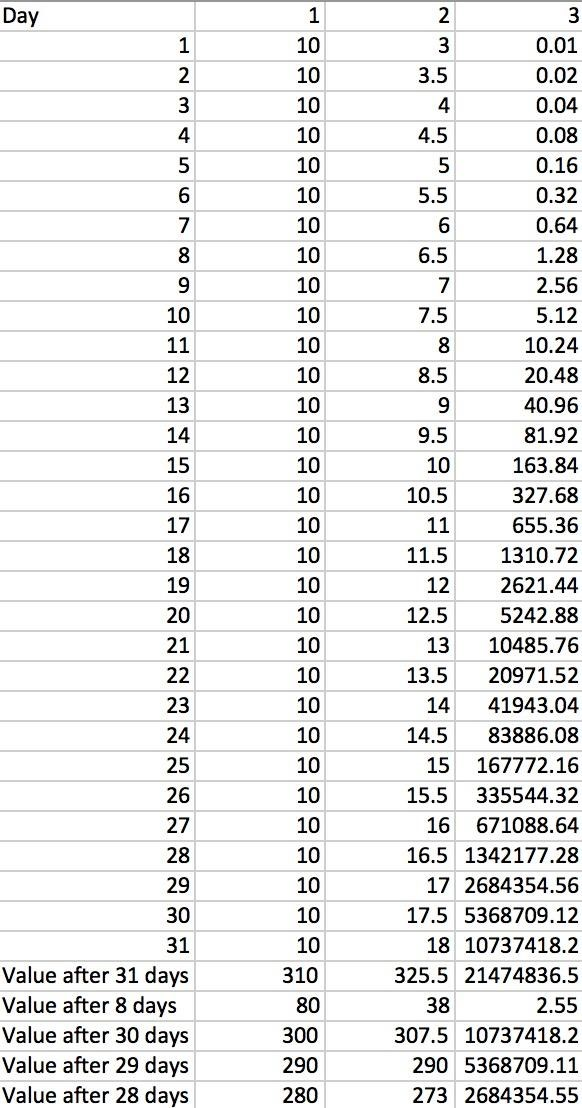Kazuma and Luanne described how they learnt about using formulae in spreadsheets, and which formulae they used:
The formula we used was a relative reference formula. We used the formula “=C2+0.5” for option 2 (so adding 0.5 to the value in the cell above) and “=D2*2” for option 3 (so multiplying the value in the cell above by 2), copying the cell values until day 31. (Note: Option 1's values stay at £10 throughout)

Anjeli used a spreadsheet with more columns to show the running totals through the month: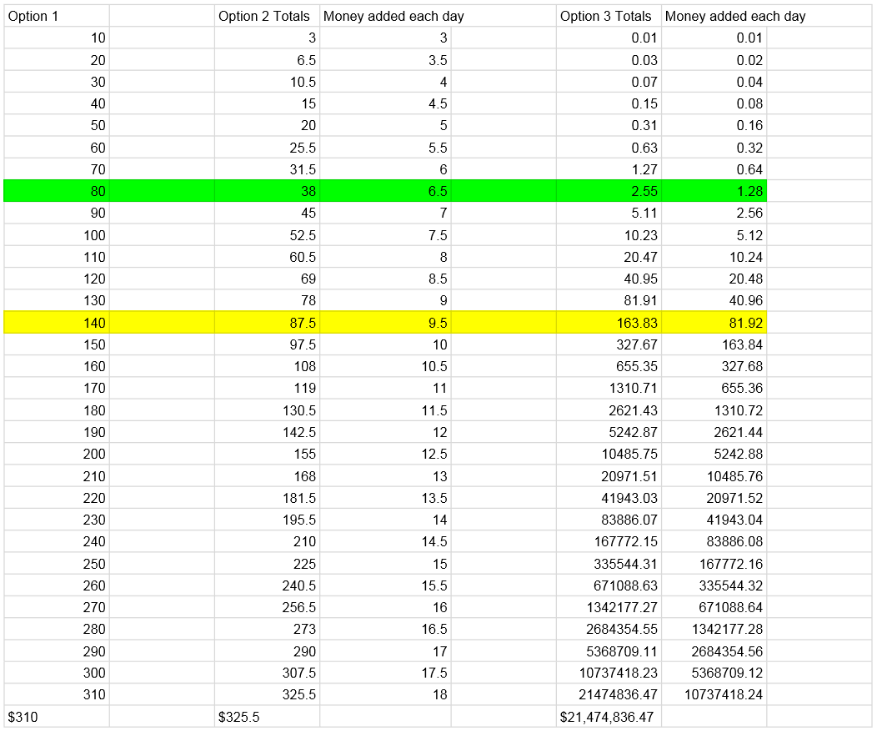Additionally, Shriya from International School Frankfurt in Germany, Jeff and Aneeka, Siya and Zara manually created tables that show the same things as these spreadsheets, and Mathias & Ashley from Peak School, Joshua, Devin (with help from Maggie) and Anana calculated some of the amounts and totals manually.

In which months would option 1 be better than option 2?
Eric, Ben, Joshua, Shriya, Ally, Clara, Kazuma and Luanne, Napat and Shawn, Jeff and James also answered this question correctly.
Clara said
:
Option 1 would be a better choice than option 2 on a February without a leap year. After that option 2 would become a lot better because every day you will be earning a lot more than 10 pounds a day.

If your family stopped your pocket money on day 8, which option would give you the most?
Mathieu, Maxim and Valentina, Eric, Ben, Shriya, Ethan and Ayaan, James, Rohan and Kazuma and Luanne answered this correctly for 8 days. Rohan said:

If pocket money stopped on day 8 option one would give you £80, option 2 £38 and option 3 only £2.55.

Joshua and Tushar considered stopping after 10 days. Tushar said:
After 10 days the sum of option 3 is: £10.23
After 10 days the sum of option 2 is: £52.5
After 10 days the sum of option 1 is: £100

On which day of the month does option 3 become the most fruitful?
Navjot pointed out that this question could be interpreted in more than one way:

The answer to this depends on what do we mean by "fruitful", do we mean when onwards will option 3 give me the most money on that particular day, or do we mean which day will our overall earnings of option 3 exceed the other options?

Some people also interpreted this question as "Which day is the best day for option 3?" rather than "On which day does option 3 become better than the other two options?", and correctly said that the best day is the last day.

A spreadsheet like Annie's, which does not show totals, is sufficient to answer Navjot's first interpretation (which is the first day when option 3 gives more money than the other options). This happens on day 11, as Ally, Jack and Clara identified.

A spreadsheet like Anjeli's is much more useful for Navjot's second interpretation (which is the first day when the total amount of money from option 3 exceeds the other totals). Kazuma and Luanne also found that this happens on day 14.

If you chose option 3, how many days would it be before you became a millionaire?
Anjeli, Shriya and Joshua used a spreadsheet or table like Anjeli's to answer this question correctly. Some people used other approaches.

Kazuma and Leanne said:
We worked backwards from day 28. We found out that you would become a millionaire on day 27.

Ally said:
If I choose option 3, it would take 27 days to become a millionaire. On the 27th day I would have £1,342,177.27. I found this out using trial and error by finding the total of different days. For example I tried the 25th day, but the total was too small so I tried the 26th day, until I found the right day.

Jeff used Hong Kong dollars instead of Great British pounds, and so got a different answer. Jeff used the exchange rate 10HKD = 1GBP.
Looking at the last column of the table, which is the accumulative frequency chart of option 3, you need to look for an amount that just passes 1000000HKD. And the answer is on day 24. Even though it is a large amount over 1 million, you have to consider that as you go down the column, the numbers that you need to add become bigger and bigger. Therefore there will be a bigger difference.

Some people said that option 3 would make you a millionaire on day 28, not day 27. Siya, Aneeka and Zara explained how this mistake could happen. Click here to see their table, which actually contains a mistake from day 23 to day 24, when a digit is lost.
We realised that the end of our first column was a total, whereas the end of the second and third colum were not. The first total was £310, the second was £331.50, and the third was obviously the winner because even without the running total, they were a millionaire!
So day 28 is the first day on which your parents would have to give you a million pounds, but by day 27 your total would have already reached £1,000,000.

Ben noticed a pattern between the running totals and the amount you receive on each day:

Let's just go through some running totals. On the second day, we get 2 pence. This is 1 pence off the total for all the days before it. There is only one day before day 2, which is day 1, where we got 1 pence. Therefore, our total is 1 pence off the next value.

Then go on to day 3. We get 4 pence on day 3, which is one pence off the total for the previous days. Our total up to this point is 1 pence + 2 pence, which is 3 pence. Notice how we have just come up with a more efficient, or better, way of working out when we will become a millionaire. Since the next number in the list is very very close to our current total, we can just call them the same. If the next number in the list is over a million, out current total is over a million and we are a millionaire! Huzzah!

Now let's look at day 27. We can use our new technique for this. So we look at the next number, or the money we will get on day 28, which is £1,342,177.28. Boom! Our current total is over £1,000,000, so we are a millionaire!

Using patterns and sequences

Amaris from German Swiss International School in Hong Kong, Juna from Dulwich College Seoul, Edward and Sylvana, Christine, Darren and Ken from German Swiss International School in Hong Kong, Andy from Redland Green School in the UK, Otis from Sandroyd in the UK, James, Ben, Annie and Navjot considered the sequences that the pocket money forms for each option. These are Ben's descriptions of the sequences:

Option 2: We have the list: $3, 3.5, 4, 4.5,$ etc etc. We can calculate the last term to get a sense of how much money we are getting. We do $0.5 \times 31,$ which is $15.5,$ and add $2.5,$ to get $18.$

Option 3: We are starting at $1$ pence, which equals $2^0$ pence. Therefore, since we are
"one behind" our position in the list [of powers of $2$] (the fifth value will be $2^4$ pence), our last value will be $2^{30}.$

So, $2^{30}$ pence $= 1073741824$ pence, and if we divide that by $100$ (to convert to pounds), we get $£10,737,418.24$. That is a LOT of pocket money!

These are Andy's descriptions of the sequences:
On option 2, there is an $N^\text{th}$ term of $£2.50 + £0.50N$

On option 3, there is an $N^\text{th}$ term of $2^{N-1}$ (in pence)

Anana, Edward, Andy, Ben and Sylvana, Christine, Darren and Ken added the amounts for option 2 correctly. Otis noticed a pattern that simplified the sum $3 + 3.5 + ...$ into a shorter sum:
I worked out that the numbers (e.g. $3+4=7$ and $5+6=11$ and if you see $11-7 =4$). This also occurs with the other sets (e.g. $3.50+4.50=8$ and $5.50+6.50=12.$  $12-8=4$). Both patterns are going up in fours in pairs.Ben considered the total amounts in option 2 by thinking about averages:
We know our minimum value is $3$ and our maximum value is $18.$ The average of this is $3 + 18 = 21,$ $21 \div 2 = 10.5.$ Therefore, we are getting a little bit more per day than we did in option 1.

Edward made a different observation about the numbers in option 2. Note that Edward worked in Hong Kong dollars, where 1GBP = 10HKD:
We start off at $\$30$, and add an extra$\$5$ per day.
2nd day: $30+5$
3rd day: $30+2(5)$
So, $n^\text{th}$ day: $30+5(n-1)$
Which can be simplified to $25 +5n$

$25n + 5 \times T(n) =$ cumulative equation
This is where triangle numbers come in, which are basically sums of numbers $\left(1+2+3+4+...\left(n-1\right)+n\right).$
To solve this, we merge the numbers into pairs, $1+n, 2+(n-1)$ and so on. They all add up to $n+1.$

This pattern goes on until we hit the median number. Therefore, the expression for triangle numbers are $\frac{n\times(n+1)}2.$

The $\frac n2$ comes in because there are $\frac n2$ pairs, if $n$ is even, and each pair adds up to $n+1$. So the sum is equal to $n+1$ added up $\frac n2$ times.

If $n$ is odd, then the middle number (the median) does not have a partner, and so there are $\frac{n-1}2$ pairs (which each add up to $n+1$). But the middle number will be $\frac{n+1}2$, so the total will be the sum of the pairs plus the middle number, which is $\frac{n-1}2\times(n+1) + \frac{n+1}2 = \frac{(n-1)(n+1) + 1(n+1)}2 = \frac{n(n+1)}2$

However, since the amount of pocket money increases by $5$ (so $5,10,15,20$ instead of $1,2,3,4$), we have to times the triangle number expression by $5$, and times the base amount of money by $n$, since we are finding the cumulative amount. Hence, we reach:
$25n + 5 \times \frac{n(n + 1)}2$

Navjot did exactly the same thing:
In option 2, I would get an extra $50$p ($£0.5$) each day, so $£3$ on day 1, $£3.50$ on day 2, $£4$ on day 3 and so on, thus being an arithmetic series.
So the series is:

$âˆ‘0.5x+2.5$ between $1â‰¤xâ‰¤31$

$= (0.5âˆ‘x) + âˆ‘2.5$

$= (0.5(31)(32)/2) + 31*2.5$

(Formula for $âˆ‘x$ to a value of $n$ is $\frac{n(n+1)}2$
$= 248 + 77.5$

$= £325.50$

Sylvana, Christine, Darren and Ken looked up some definitions and formulae to find the sum:
For the second sequence, the numbers/money received each day increases by a common difference, which is $0.5$ dollars. It is an arithmetic sequence.

The equation for the total sum of an arithmetic sequence is (where from?) $$S_n=\frac n2\left(a_1 + a_n\right)$$
This formula can actually be derived in the same way that Edward derived the formula for finding the triangular numbers.

However, we do not know what "$a_n$" is, and we must input another equation into this formula. "$a_n$" can be expressed as $a_n=a_1+(n-1)d$.

By inputting this equation in "$S_n$", we can find the sum of the sequence with the equation $$S_n = \frac n2 \left(2a_1 + \left(n-1\right)d\right)$$ (in this case, $n$ being the total number of days ($30$), $a_1$ being the first number/amount ($3$) and $d$ being the common difference ($0.5$))

So therefore, $S_n = \frac{30}2 \left( 2\left(3\right) + \left(30-1\right)0.5\right)$, which gives $307.5$ pounds after $30$ days. This equation can be used to calculate the sum of money after any given day.

Edward, Navjot and Sylvana, Christine, Darren and Ken successfully found the total pocket money for option 3. This is Edward's work:
Boiling down this problem, it comes down to this: what is the triangle number for $2^0+2^1+2^2+...+2^n$?$2^0 + 2^1 + 2^2 + 2^3 + ... + 2^{n-1} = 2^n-1$

Why is this, you may ask.

Looking at Edward's sums, you might notice that each time Edward adds a new term, he does the sum so far, plus the next term, so $(2^k-1) + 2^{(k+1)-1}$, which is equal to $2^k-1+2^k$, which is equal to $2\times2^k-1=2^{k+1}-1$. So the pattern will continue.

Edward used a different technique to prove that the pattern continues:

Let us first look at summing up powers of $2$ in binary. Each power of $2$ is a $1$ followed by zeroes ($1=1, 2=10, 4=100, 8=1000$ etc). As you move on to the next power, you add one more zero. $2^n$ will be a $1$ followed by $n$ zeroes. This means that when you sum up the powers of $2$, you get a string of $1$s:
$1+2+4=7=1+10+100=111,$      $1+2+4+8=1+10+100+1000=1111.$

Such strings are always $1$ less than a power of $2$. If you add $1$ to such a string, you will get a $1$ followed by $n+1$ zeroes (where $n$ is the power you are summing up to).

Because you start at day $1$, not day $0$, $n$ becomes $n-1$ so $2^{n+1}$ becomes $2^n$

Sylvana, Christine, Darren and Ken and Navjot used another formula. This is Navjot's work:
As the amount I get doubles each day, my series would be a geometric one:
$âˆ‘2^{x-1}$ between $1â‰¤xâ‰¤31$

The formula for a finite geometric sequence is $\frac{a(r^n-1)}{r-1}$ where $a$ is the first term of the series, $r$ is the common ratio, and $n$ is the final $x$ value of the series.

In this case, $a=1$ since we start with 1p, $r$ is $2$ since the amount I get doubles every day, and $n$ is $31,$ the number of days in the month.

Therefore, the series adds up to:
$\frac{1(2^{31}-1)}{2-1} = 2^{31}-1 = 2147483647\text pâ‰ˆ 2.1\times10^9\text p= £2.1\times10^7$

Navjot used the same formulae to answer the next few questions:
1) In which months would option 1 be better than option 2?
Months will have $28, 29, 30$ or $31$ days

In February (non leap year, $28$ days):
Option 1 would give me $£10\times28 = £280$
Option 2 would give me $0.5(28)(29)\div2+2.5(28)= £273$
Therefore option 1 is better in a non leap year February by $£7.$

In February (leap year, $29$ days):
Option 1 would give me $£10\times29 = £290$
Option 2 would give me $0.5(29)(30)\div2+2.5(29)= £290$
Therefore, both the options will give me the same amount in a leap year February.

In a $30$ day month:
Option 1 would give me $£10\times30 = £300$
Option 2 would give me $0.5(30)(31)\div2+2.5(30)= £307.5$
Therefore, option 2 is better in a $30$ day month by $£7.5$

2) If your family stopped your pocket money on day 8, which option would give you the most?

Option 1 would give me $8\times £10 = £80$
Option 2 would give me $0.5(8)(9)\div2 +2.5(8)= £38$
Option 3 would give me $2^8-1 = 255\text p = £2.55$

Option 1 would be a lot better in a span of $8$ days.

4) If you chose option 3, how many days would it be before you became a millionaire?

$£1,000,000 = 100,000,000\text p$
Therefore, $âˆ‘2^{x-1} > 100000000$
(When the summation happens between the interval $1â‰¤xâ‰¤n$, $n$ being the day the sum satisfies the inequality)

Or, $$2^x-1 > 10000000\\ 2^x > 100000001\\ \log_2{100000001} > x\\$$
Therefore, $26.5754$ ($\log_2{100000001}=26.5754$)

This means that I would become a millionaire on the 27th day.

Graphs

Amaris, James and Navjot used graphs to investigate the problem. It is unclear how Amaris generated the graph below, but it shows the cumulative (running total) amount of money earned for each of the three options (in Hong Kong dollars).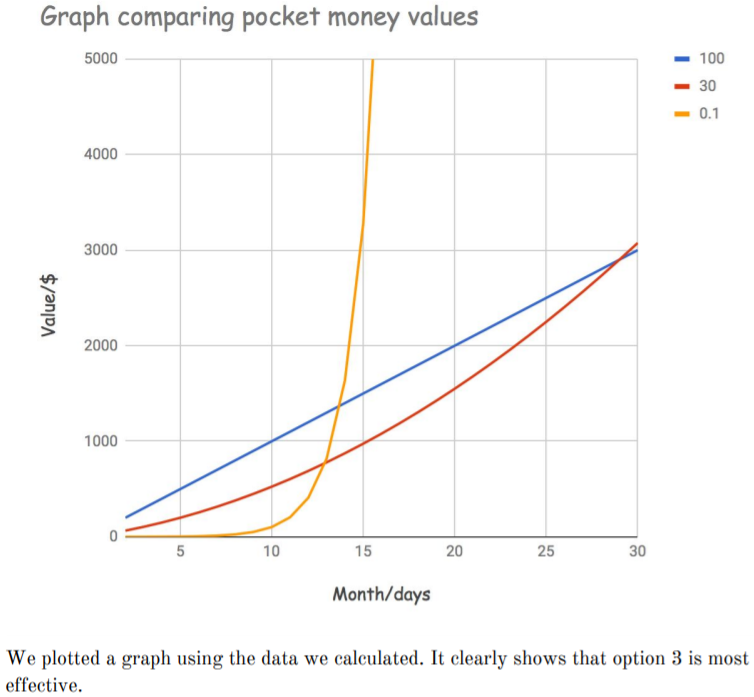James used Excel to plot a graph for the first 8 days. James' graph shows the amount of money earned per day, as well as the cumulative amounts of money (running totals):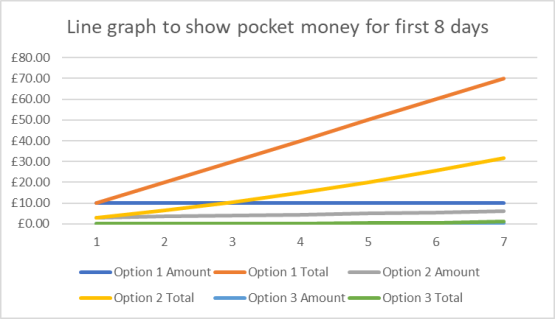For the scale of this graph, Option 3 Amount and Option 3 Total are both so small that they can't really be seen as two distinct lines, and appear on top of each other.

Navjot explored when option 3 becomes the most fruitful option, in terms of both when it becomes the option which gives most money each day, and when option 3 becomes the option which has given more money in total.

If the former is the intended question, the following will be the inequalities:

1)  $2^{x-1}>10$, (Option 3 vs. 1)
2)  $2^{x-1}>0.5x+2.5$, (Option 3 vs. 2)

Navjot has forgotten to convert between pounds and pence, so option 3 is expressed in pence, but options 1 and 2 are expressed in pounds. This leads to incorrect answers, but Navjot's method is not incorrect.

I was unable to solve these inequalities algebraically, so I used desmos.com to answer them graphically.
We were unable to open Navjot's graphs, so have recreated them using desmos.com.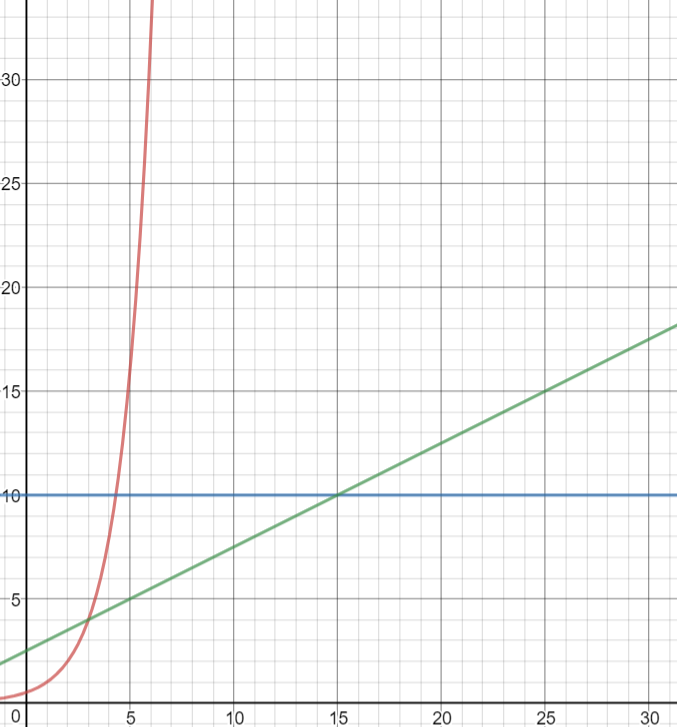The red curve is $y=2^{x-1}$
The blue line is $y=10$
And the green line is $y=0.5x+2.5$

As we can see, the red curve crosses the green line at $x=3,$ which means that after the 4th day option 3 will be more fruitful per day than option 2.

Also, the red curve crosses the blue line at $x=4.322,$ which means that on the 5th day, option 3 will become more fruitful per day than option 1, becoming the most fruitful option per day after that.

If the point of the question was to find the day when the overall earnings from option 3 would exceed the other 2 options, the inequalities would be as follows:

1)  $2^x-1>10x$, (Option 3 vs. 1)
2)  $2^x-1>0.5\times x(x+1)\div2 +2.5x$, (Option 3 vs. 2)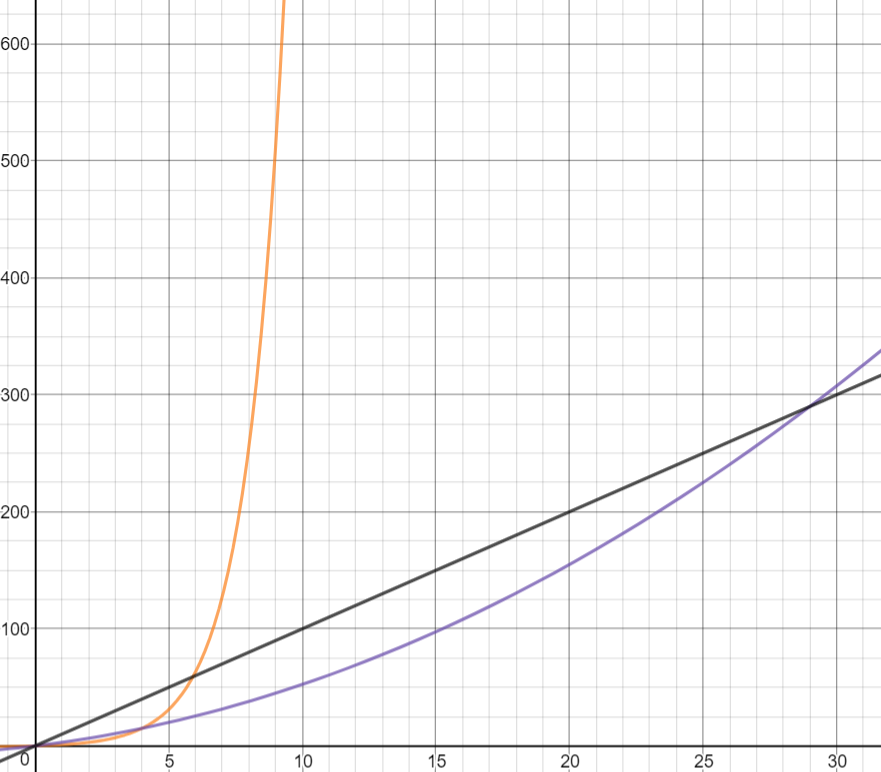I was unable to solve these algebraically either, therefore I used desmos.com again to solve these graphically.

In the second graph:
The orange curve is $y=2^x-1,$
The black curve is $y=10x$
And, the blue curve is $y=0.5\times x(x+1)\div2 +2.5x$

As shown in the graph, the orange curve crosses the blue curve first at $x=4,$ so option 3 will become better than option 2 on the 4th day.

It also crosses the black line at $x=5.909,$ which means that it will become a better option that option 1 on the 6th day, becoming the best option overall after that.

Well done to Anthony, Brian and Justin from German Swiss International School in Hong Kong, whose investigation used all of the approaches desribed above. Click here to see their work.